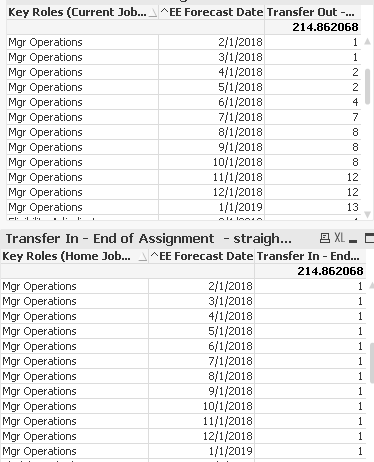# QlikView App Development

Discussion Board for collaboration related to QlikView App Development.

Highlighted
New Contributor

## When Two Sums () based on Two Fields/Dimensions, How to combine them into one straight table

The two straight tables have three columns - first two columns are dimensions and the third is a Sum () expression

• The first column (dimension) in two tables come from TWO DIFFERENT  fields in the same load statement
• These two different fields contain same values
• But most rows in the load statement may have different values in the load statement.
• e.g. one row may have ABC and ABC in two fields; one row may have ABC and XYZ
• The second column (dimension) in two tables come from SAME filed in the same load statement
• the third column (Sum () expression) is based on the 1st and 2nd column.My questions is how to incorporate two SUM () columns into one single straight table

• a straight table has four columns
• two dimensions (Roles and Date)
• 3rd column is Transfer Out (e.g. row values = 1, 1,2,2,4,7,8,...) (the 3rd column in the first table above)
• 4th column is Transfer In (e.g. row values = 1,1,1,1,1,.....)   (the 3rd column in the second table above)
Tags (3)